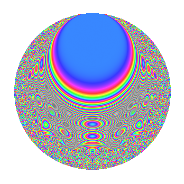# Properties

 Label 8047.2.a.dLevel 8047 Weight 2 Character orbit 8047.a Self dual Yes Analytic conductor 64.256 Analytic rank 0 Dimension 156 CM No

# Related objects

## Newspace parameters

 Level: $$N$$ = $$8047 = 13 \cdot 619$$ Weight: $$k$$ = $$2$$ Character orbit: $$[\chi]$$ = 8047.a (trivial)

## Newform invariants

 Self dual: Yes Analytic conductor: $$64.2556185065$$ Analytic rank: $$0$$ Dimension: $$156$$ Fricke sign: $$-1$$ Sato-Tate group: $\mathrm{SU}(2)$

## $q$-expansion

The dimension is sufficiently large that we do not compute an algebraic $$q$$-expansion, but we have computed the trace expansion.

 $$\operatorname{Tr}(f)(q) =$$ $$156q$$ $$\mathstrut +\mathstrut 13q^{2}$$ $$\mathstrut +\mathstrut 23q^{3}$$ $$\mathstrut +\mathstrut 161q^{4}$$ $$\mathstrut +\mathstrut 39q^{5}$$ $$\mathstrut +\mathstrut 25q^{6}$$ $$\mathstrut +\mathstrut 19q^{7}$$ $$\mathstrut +\mathstrut 42q^{8}$$ $$\mathstrut +\mathstrut 169q^{9}$$ $$\mathstrut +\mathstrut O(q^{10})$$ $$\operatorname{Tr}(f)(q) =$$ $$156q$$ $$\mathstrut +\mathstrut 13q^{2}$$ $$\mathstrut +\mathstrut 23q^{3}$$ $$\mathstrut +\mathstrut 161q^{4}$$ $$\mathstrut +\mathstrut 39q^{5}$$ $$\mathstrut +\mathstrut 25q^{6}$$ $$\mathstrut +\mathstrut 19q^{7}$$ $$\mathstrut +\mathstrut 42q^{8}$$ $$\mathstrut +\mathstrut 169q^{9}$$ $$\mathstrut +\mathstrut 11q^{10}$$ $$\mathstrut +\mathstrut 23q^{11}$$ $$\mathstrut +\mathstrut 57q^{12}$$ $$\mathstrut -\mathstrut 156q^{13}$$ $$\mathstrut +\mathstrut 18q^{14}$$ $$\mathstrut +\mathstrut 32q^{15}$$ $$\mathstrut +\mathstrut 159q^{16}$$ $$\mathstrut +\mathstrut 119q^{17}$$ $$\mathstrut +\mathstrut 36q^{18}$$ $$\mathstrut +\mathstrut 35q^{19}$$ $$\mathstrut +\mathstrut 109q^{20}$$ $$\mathstrut +\mathstrut 33q^{21}$$ $$\mathstrut +\mathstrut 11q^{22}$$ $$\mathstrut +\mathstrut 55q^{23}$$ $$\mathstrut +\mathstrut 63q^{24}$$ $$\mathstrut +\mathstrut 189q^{25}$$ $$\mathstrut -\mathstrut 13q^{26}$$ $$\mathstrut +\mathstrut 89q^{27}$$ $$\mathstrut +\mathstrut 54q^{28}$$ $$\mathstrut -\mathstrut 55q^{29}$$ $$\mathstrut +\mathstrut 47q^{31}$$ $$\mathstrut +\mathstrut 112q^{32}$$ $$\mathstrut +\mathstrut 109q^{33}$$ $$\mathstrut +\mathstrut 51q^{34}$$ $$\mathstrut +\mathstrut 25q^{35}$$ $$\mathstrut +\mathstrut 162q^{36}$$ $$\mathstrut +\mathstrut 53q^{37}$$ $$\mathstrut +\mathstrut 37q^{38}$$ $$\mathstrut -\mathstrut 23q^{39}$$ $$\mathstrut +\mathstrut 25q^{40}$$ $$\mathstrut +\mathstrut 113q^{41}$$ $$\mathstrut +\mathstrut 26q^{42}$$ $$\mathstrut +\mathstrut 31q^{43}$$ $$\mathstrut +\mathstrut 86q^{44}$$ $$\mathstrut +\mathstrut 144q^{45}$$ $$\mathstrut +\mathstrut 37q^{46}$$ $$\mathstrut +\mathstrut 115q^{47}$$ $$\mathstrut +\mathstrut 129q^{48}$$ $$\mathstrut +\mathstrut 189q^{49}$$ $$\mathstrut +\mathstrut 72q^{50}$$ $$\mathstrut -\mathstrut 4q^{51}$$ $$\mathstrut -\mathstrut 161q^{52}$$ $$\mathstrut +\mathstrut 51q^{53}$$ $$\mathstrut +\mathstrut 108q^{54}$$ $$\mathstrut +\mathstrut 22q^{55}$$ $$\mathstrut +\mathstrut 39q^{56}$$ $$\mathstrut +\mathstrut 102q^{57}$$ $$\mathstrut +\mathstrut 31q^{58}$$ $$\mathstrut +\mathstrut 75q^{59}$$ $$\mathstrut +\mathstrut 97q^{60}$$ $$\mathstrut +\mathstrut 7q^{61}$$ $$\mathstrut +\mathstrut 77q^{62}$$ $$\mathstrut +\mathstrut 94q^{63}$$ $$\mathstrut +\mathstrut 158q^{64}$$ $$\mathstrut -\mathstrut 39q^{65}$$ $$\mathstrut +\mathstrut 48q^{66}$$ $$\mathstrut +\mathstrut 37q^{67}$$ $$\mathstrut +\mathstrut 235q^{68}$$ $$\mathstrut +\mathstrut 27q^{69}$$ $$\mathstrut +\mathstrut 38q^{70}$$ $$\mathstrut +\mathstrut 70q^{71}$$ $$\mathstrut +\mathstrut 152q^{72}$$ $$\mathstrut +\mathstrut 155q^{73}$$ $$\mathstrut -\mathstrut 18q^{74}$$ $$\mathstrut +\mathstrut 80q^{75}$$ $$\mathstrut +\mathstrut 21q^{76}$$ $$\mathstrut +\mathstrut 101q^{77}$$ $$\mathstrut -\mathstrut 25q^{78}$$ $$\mathstrut +\mathstrut 10q^{79}$$ $$\mathstrut +\mathstrut 211q^{80}$$ $$\mathstrut +\mathstrut 220q^{81}$$ $$\mathstrut +\mathstrut 45q^{82}$$ $$\mathstrut +\mathstrut 132q^{83}$$ $$\mathstrut +\mathstrut 86q^{84}$$ $$\mathstrut +\mathstrut 74q^{85}$$ $$\mathstrut +\mathstrut 35q^{86}$$ $$\mathstrut +\mathstrut 53q^{87}$$ $$\mathstrut +\mathstrut 51q^{88}$$ $$\mathstrut +\mathstrut 190q^{89}$$ $$\mathstrut -\mathstrut 27q^{90}$$ $$\mathstrut -\mathstrut 19q^{91}$$ $$\mathstrut +\mathstrut 125q^{92}$$ $$\mathstrut +\mathstrut 96q^{93}$$ $$\mathstrut -\mathstrut 19q^{94}$$ $$\mathstrut +\mathstrut 72q^{95}$$ $$\mathstrut +\mathstrut 146q^{96}$$ $$\mathstrut +\mathstrut 155q^{97}$$ $$\mathstrut +\mathstrut 135q^{98}$$ $$\mathstrut +\mathstrut 89q^{99}$$ $$\mathstrut +\mathstrut O(q^{100})$$

## Embeddings

For each embedding $$\iota_m$$ of the coefficient field, the values $$\iota_m(a_n)$$ are shown below.

For more information on an embedded modular form you can click on its label.

Label $$a_{2}$$ $$a_{3}$$ $$a_{4}$$ $$a_{5}$$ $$a_{6}$$ $$a_{7}$$ $$a_{8}$$ $$a_{9}$$ $$a_{10}$$
1.1 −2.78003 2.35788 5.72856 3.19176 −6.55498 −2.60821 −10.3655 2.55961 −8.87319
1.2 −2.75185 0.388525 5.57269 0.235747 −1.06916 −0.913788 −9.83151 −2.84905 −0.648741
1.3 −2.71116 −0.430887 5.35037 1.49097 1.16820 4.38752 −9.08338 −2.81434 −4.04226
1.4 −2.66092 −2.09208 5.08047 4.25784 5.56685 −2.19372 −8.19688 1.37680 −11.3297
1.5 −2.65345 0.515937 5.04077 −0.00500829 −1.36901 0.475978 −8.06853 −2.73381 0.0132892
1.6 −2.61991 −3.07843 4.86391 −2.25474 8.06519 3.02795 −7.50318 6.47672 5.90721
1.7 −2.53163 −1.76740 4.40915 3.70059 4.47441 1.97275 −6.09908 0.123713 −9.36852
1.8 −2.51520 3.16825 4.32624 1.81160 −7.96880 1.29182 −5.85097 7.03782 −4.55654
1.9 −2.51030 2.30230 4.30163 −2.11540 −5.77947 3.11614 −5.77778 2.30059 5.31029
1.10 −2.48921 1.66409 4.19617 0.253243 −4.14227 −2.12862 −5.46674 −0.230804 −0.630376
1.11 −2.43558 −1.59767 3.93207 −2.50698 3.89125 −1.32806 −4.70573 −0.447465 6.10595
1.12 −2.42534 −2.30091 3.88226 −2.29855 5.58047 −0.451041 −4.56512 2.29416 5.57476
1.13 −2.42132 −1.26535 3.86281 0.549340 3.06382 1.75655 −4.51045 −1.39889 −1.33013
1.14 −2.40103 1.80838 3.76492 2.80011 −4.34197 −2.97647 −4.23763 0.270237 −6.72314
1.15 −2.37112 0.716095 3.62222 −3.83331 −1.69795 −1.71381 −3.84647 −2.48721 9.08923
1.16 −2.35417 1.10999 3.54214 −1.30122 −2.61310 −1.47122 −3.63046 −1.76793 3.06330
1.17 −2.22460 3.13495 2.94885 −1.36986 −6.97401 0.348323 −2.11081 6.82790 3.04739
1.18 −2.19459 1.61581 2.81621 −2.64713 −3.54605 3.12381 −1.79125 −0.389142 5.80935
1.19 −2.16081 −2.05453 2.66911 −1.91838 4.43946 −4.69995 −1.44582 1.22110 4.14525
1.20 −2.15517 0.169019 2.64475 4.16569 −0.364265 2.92802 −1.38954 −2.97143 −8.97775
See next 80 embeddings (of 156 total)
 $$n$$: e.g. 2-40 or 990-1000 Embeddings: e.g. 1-3 or 1.156 Significant digits: Format: Complex embeddings Normalized embeddings Satake parameters Satake angles

## Inner twists

This newform does not have CM; other inner twists have not been computed.

## Atkin-Lehner signs

$$p$$ Sign
$$13$$ $$1$$
$$619$$ $$-1$$

## Hecke kernels

This newform can be constructed as the kernel of the linear operator $$T_{2}^{156} - \cdots$$ acting on $$S_{2}^{\mathrm{new}}(\Gamma_0(8047))$$.